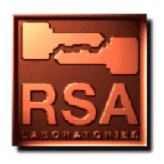quantum parallelism;destructive interference phenomena;algorithms">### Question 109. What is Quantum Computing?

Quantum computing [Ben82][Fey82] [Fey86][Deu92] is a new field in computer science that has been developed with our increased understanding of quantum mechanics. It holds the key to computers that are exponentially faster than conventional computers (for certain problems). A quantum computer is based on the idea of a quantum bit or qubit. In classical computers, the bit has a discrete range and can represent either a zero or a one. A qubit can be in a linear superposition of the two states. A quantum register consists of n qubits. Because of superposition, a phenomenon known as quantum parallelism allows exponentially many such computations to take place simultaneously, thus vastly increasing the speed of computation.

Quantum interference, the analog of Young's double-slit experiment which demonstrated constructive and destructive interference phenomena of light, is one of the most significant characteristics of quantum computing. Quantum interference improves the probability of obtaining a desired result by constructive interference and diminishes the probability of obtaining an erroneous result by destructive interference. Thus, among the exponentially many computations, the correct answer can theoretically be identified with appropriate quantum "algorithms."

It has been proven [Sho94] that a quantum computer will be able to factor (see Question 45) and compute discrete logarithms (see Question 52) in polynomial time. Unfortunately, the development of a practical quantum computer still seems far away because of a phenomenon called quantum decoherence, which is due to the influence of the outside environment on the quantum computer. Brassard has written a number of helpful texts in this field [Bra95a][Bra95b] [Bra95c].

Quantum cryptography (see Question 110) is quite different from, and currently more viable than, quantum computing.